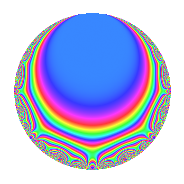# Properties

 Label 8016.2.a.rLevel 8016 Weight 2 Character orbit 8016.a Self dual Yes Analytic conductor 64.008 Analytic rank 1 Dimension 5 CM No Inner twists 1

# Related objects

## Newspace parameters

 Level: $$N$$ = $$8016 = 2^{4} \cdot 3 \cdot 167$$ Weight: $$k$$ = $$2$$ Character orbit: $$[\chi]$$ = 8016.a (trivial)

## Newform invariants

 Self dual: Yes Analytic conductor: $$64.0080822603$$ Analytic rank: $$1$$ Dimension: $$5$$ Coefficient field: 5.5.11256624.1 Coefficient ring: $$\Z[a_1, \ldots, a_{13}]$$ Coefficient ring index: $$1$$ Fricke sign: $$1$$ Sato-Tate group: $\mathrm{SU}(2)$

## $q$-expansion

Coefficients of the $$q$$-expansion are expressed in terms of a basis $$1,\beta_1,\beta_2,\beta_3,\beta_4$$ for the coefficient ring described below. We also show the integral $$q$$-expansion of the trace form.

 $$f(q)$$ $$=$$ $$q - q^{3} + \beta_{2} q^{5} + ( -2 + \beta_{4} ) q^{7} + q^{9} +O(q^{10})$$ $$q - q^{3} + \beta_{2} q^{5} + ( -2 + \beta_{4} ) q^{7} + q^{9} -\beta_{3} q^{11} + ( 1 + \beta_{1} ) q^{13} -\beta_{2} q^{15} + ( 1 - \beta_{1} ) q^{17} + ( -2 + \beta_{3} ) q^{19} + ( 2 - \beta_{4} ) q^{21} + \beta_{3} q^{23} + ( 3 - 2 \beta_{1} + \beta_{4} ) q^{25} - q^{27} + ( -2 + 2 \beta_{1} - \beta_{3} ) q^{29} + ( -2 - \beta_{3} - \beta_{4} ) q^{31} + \beta_{3} q^{33} + ( -\beta_{2} + \beta_{3} - 2 \beta_{4} ) q^{35} + ( 1 + \beta_{1} - \beta_{2} + \beta_{3} ) q^{37} + ( -1 - \beta_{1} ) q^{39} + ( 1 - \beta_{1} - 2 \beta_{2} + \beta_{3} ) q^{41} + ( -4 + 2 \beta_{1} - \beta_{3} - 2 \beta_{4} ) q^{43} + \beta_{2} q^{45} + ( 2 - 2 \beta_{1} - \beta_{4} ) q^{47} + ( 3 - 2 \beta_{2} - \beta_{3} - 3 \beta_{4} ) q^{49} + ( -1 + \beta_{1} ) q^{51} + ( -3 \beta_{2} - \beta_{3} - 2 \beta_{4} ) q^{53} + ( 2 - 2 \beta_{1} + \beta_{3} - 2 \beta_{4} ) q^{55} + ( 2 - \beta_{3} ) q^{57} + ( -3 + 3 \beta_{1} + \beta_{2} + \beta_{3} + 2 \beta_{4} ) q^{59} + ( 4 - 2 \beta_{1} - 2 \beta_{4} ) q^{61} + ( -2 + \beta_{4} ) q^{63} + ( -2 + 2 \beta_{1} + \beta_{3} ) q^{65} + ( -2 + \beta_{2} - 2 \beta_{3} - 2 \beta_{4} ) q^{67} -\beta_{3} q^{69} + ( 2 - 2 \beta_{1} - 2 \beta_{2} + \beta_{3} + 2 \beta_{4} ) q^{71} + ( 2 + 2 \beta_{2} + \beta_{3} + 2 \beta_{4} ) q^{73} + ( -3 + 2 \beta_{1} - \beta_{4} ) q^{75} + ( 2 - 2 \beta_{1} - 2 \beta_{2} + 3 \beta_{3} + 2 \beta_{4} ) q^{77} + ( -1 - \beta_{1} - 2 \beta_{2} + \beta_{3} ) q^{79} + q^{81} + ( -1 + \beta_{1} + \beta_{2} + 4 \beta_{4} ) q^{83} + ( 2 - 2 \beta_{1} + 2 \beta_{2} - \beta_{3} ) q^{85} + ( 2 - 2 \beta_{1} + \beta_{3} ) q^{87} + ( -2 + 2 \beta_{1} + 3 \beta_{4} ) q^{89} + ( -2 - 2 \beta_{1} + \beta_{3} + 2 \beta_{4} ) q^{91} + ( 2 + \beta_{3} + \beta_{4} ) q^{93} + ( -2 + 2 \beta_{1} - 2 \beta_{2} - \beta_{3} + 2 \beta_{4} ) q^{95} + ( -2 + 4 \beta_{1} + 2 \beta_{2} + \beta_{4} ) q^{97} -\beta_{3} q^{99} +O(q^{100})$$ $$\operatorname{Tr}(f)(q)$$ $$=$$ $$5q - 5q^{3} - q^{5} - 9q^{7} + 5q^{9} + O(q^{10})$$ $$5q - 5q^{3} - q^{5} - 9q^{7} + 5q^{9} + 2q^{11} + 6q^{13} + q^{15} + 4q^{17} - 12q^{19} + 9q^{21} - 2q^{23} + 14q^{25} - 5q^{27} - 6q^{29} - 9q^{31} - 2q^{33} - 3q^{35} + 5q^{37} - 6q^{39} + 4q^{41} - 18q^{43} - q^{45} + 7q^{47} + 16q^{49} - 4q^{51} + 3q^{53} + 4q^{55} + 12q^{57} - 13q^{59} + 16q^{61} - 9q^{63} - 10q^{65} - 9q^{67} + 2q^{69} + 10q^{71} + 8q^{73} - 14q^{75} + 6q^{77} - 6q^{79} + 5q^{81} - q^{83} + 8q^{85} + 6q^{87} - 5q^{89} - 12q^{91} + 9q^{93} - 2q^{95} - 7q^{97} + 2q^{99} + O(q^{100})$$

Basis of coefficient ring in terms of a root $$\nu$$ of $$x^{5} - x^{4} - 16 x^{3} + 20 x^{2} + 31 x - 11$$:

 $$\beta_{0}$$ $$=$$ $$1$$ $$\beta_{1}$$ $$=$$ $$\nu$$ $$\beta_{2}$$ $$=$$ $$($$$$\nu^{2} - 7$$$$)/2$$ $$\beta_{3}$$ $$=$$ $$($$$$\nu^{3} + \nu^{2} - 11 \nu - 3$$$$)/2$$ $$\beta_{4}$$ $$=$$ $$($$$$\nu^{4} - 14 \nu^{2} + 8 \nu + 17$$$$)/4$$
 $$1$$ $$=$$ $$\beta_0$$ $$\nu$$ $$=$$ $$\beta_{1}$$ $$\nu^{2}$$ $$=$$ $$2 \beta_{2} + 7$$ $$\nu^{3}$$ $$=$$ $$2 \beta_{3} - 2 \beta_{2} + 11 \beta_{1} - 4$$ $$\nu^{4}$$ $$=$$ $$4 \beta_{4} + 28 \beta_{2} - 8 \beta_{1} + 81$$

## Embeddings

For each embedding $$\iota_m$$ of the coefficient field, the values $$\iota_m(a_n)$$ are shown below.

For more information on an embedded modular form you can click on its label.

Label $$\iota_m(\nu)$$ $$a_{2}$$ $$a_{3}$$ $$a_{4}$$ $$a_{5}$$ $$a_{6}$$ $$a_{7}$$ $$a_{8}$$ $$a_{9}$$ $$a_{10}$$
1.1
 0.308735 −1.14410 2.50259 3.20970 −3.87693
0 −1.00000 0 −3.45234 0 2.53613 0 1.00000 0
1.2 0 −1.00000 0 −2.84552 0 −4.19124 0 1.00000 0
1.3 0 −1.00000 0 −0.368511 0 −4.85901 0 1.00000 0
1.4 0 −1.00000 0 1.65109 0 −0.854515 0 1.00000 0
1.5 0 −1.00000 0 4.01528 0 −1.63136 0 1.00000 0
 $$n$$: e.g. 2-40 or 990-1000 Embeddings: e.g. 1-3 or 1.5 Significant digits: Format: Complex embeddings Normalized embeddings Satake parameters Satake angles

## Inner twists

This newform does not admit any (nontrivial) inner twists.

## Atkin-Lehner signs

$$p$$ Sign
$$2$$ $$-1$$
$$3$$ $$1$$
$$167$$ $$-1$$

## Hecke kernels

This newform can be constructed as the intersection of the kernels of the following linear operators acting on $$S_{2}^{\mathrm{new}}(\Gamma_0(8016))$$:

 $$T_{5}^{5} + T_{5}^{4} - 19 T_{5}^{3} - 21 T_{5}^{2} + 60 T_{5} + 24$$ $$T_{7}^{5} + 9 T_{7}^{4} + 15 T_{7}^{3} - 49 T_{7}^{2} - 132 T_{7} - 72$$ $$T_{11}^{5} - 2 T_{11}^{4} - 28 T_{11}^{3} + 60 T_{11}^{2} + 144 T_{11} - 288$$ $$T_{13}^{5} - 6 T_{13}^{4} - 2 T_{13}^{3} + 52 T_{13}^{2} - 48 T_{13} - 8$$